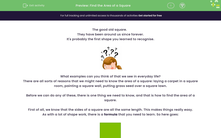# Find the Area of a Square

In this worksheet, students will learn the formula for finding the area of a square and use it to find areas.Key stage:  KS 4

Year:  GCSE

GCSE Subjects:   Maths

GCSE Boards:   AQA, Eduqas, Pearson Edexcel, OCR,

Curriculum topic:   Geometry and Measures, Mensuration

Curriculum subtopic:   Mensuration and Calculation Area Calculations

Popular topics:   Area worksheets, Geometry worksheets

Difficulty level:#### Worksheet Overview

The good old square.

They have been around us since forever.

It's probably the first shape you learned to recognise.What examples can you think of that we see in everyday life?

There are all sorts of reasons that we might need to know the area of a square: laying a carpet in a square room, painting a square wall, putting grass seed over a square lawn.

Before we can do any of these, there is one thing we need to know, and that is how to find the area of a square.

First of all, we know that the sides of a square are all the same length. This makes things really easy.

As with a lot of shape work, there is a formula that you need to learn. So here goes:Area of a square = base x height

Yes, really that is all there is to it.

Find the area of this square:Hang on, I only have the length of one side.

Of course, the sides of a square are all the same.

Area = base x height

Area = 62 x 62 = 3844 mm²

As we are working with area, units are always squared. e.g cm²,  m²

There will be no stopping you now!

### What is EdPlace?

We're your National Curriculum aligned online education content provider helping each child succeed in English, maths and science from year 1 to GCSE. With an EdPlace account you’ll be able to track and measure progress, helping each child achieve their best. We build confidence and attainment by personalising each child’s learning at a level that suits them.

Get started